# Cellular Automata Algorithms

A cellular automaton is a formal device consisting of

• a grid structure, itself consisting of cells, which may be occupied (or not) by items which possess one or more properties and
• a rule or set of rules which determine how a pattern of occupied cells is succeeded by a new pattern at the next step.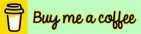This page contains algorithms for five cellular automata. What is common to all five is as follows:

1. A rectangular grid whose elements (positions) are specified by row number and column number.
2. Each position in the grid is associated with a certain state, which is specified by a number. Each position is said to be occupied by a cell in a certain state, or alternatively a position with associated state zero may be said to be "empty", the other positions being occupied by cells in some (non-zero) state.
3. A set of rules which determines how the state of a cell (or of a position in the grid) changes from one "step" or "generation" to the next, usually as a function of the state of the cells (if any) around it and its own state.
4. Periodic boundary conditions.

Thus from an initial state or configuration of the grid we obtain a series of states or configurations, and thus the cellular automaton may be thought of as evolving over time (or as traversing a path through the "state space").

These cellular automata have been implemented by the author in his program Five Cellular Automata. The images at right show snapshots of the dynamic output of this program.

The user interface for this program is written in Visual Basic 6. To run the cellular automata this program calls functions in a DLL written in C. The source code for these functions is given here:

### q-state Life

The rules for q-state Life are as follows: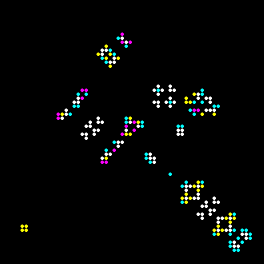(i) Select four integers k1, k2, k3 and k4 in the range 0 through 900. (ii) In the transition from one step to the next the state of each cell is changed once according to rules (iii) - (v). (iii) For each cell let S denote the sum of the states of its eight neighbors (S does not include the state of the cell itself). (iv) Let s denote the state of the cell. If s > q/2 then if k1 <= S <= k2 then add 1 (if s < q) to the state of the cell, otherwise subtract 1 (if s > 1). (v) Otherwise (i.e. if s <= q/2) if k3 <= S <= k4 then add 1 (if s < q) to the state of the cell, otherwise subtract 1 (if s > 1).

Taking q = 2, k1 = 10 and k2, k3, k4 each equal to 11 we obtain rules which are equivalent to the rules for Conway's Life (taking 'dead' = state 1 and 'alive' = state 2).

### The Belousov-Zhabotinsky Reaction

The rules, as given by Professor A. K. Dewdney, to be applied to a cell, in order to determine its state in the next generation, are as follows: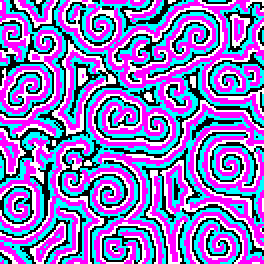(i) If the cell is healthy (i.e., in state 0) then its new state is [a/k1] + [b/k2], where a is the number of infected cells among its eight neighbors, b is the number of ill cells among its neighbors, and k1 and k2 are constants. Here "[]" means the integer part of the number enclosed, so that, for example, [7/3] = [2+1/3] = 2. (ii) If the cell is ill (i.e., in state n) then it miraculously becomes healthy (i.e., its state becomes 0). (iii) If the cell is infected (i.e., in a state other than 0 and n) then its new state is [s/(a+b+1)] + g, where a and b are as above, s is the sum of the states of the cell and of its neighbors and g is a constant.

Rule (iii) can be seen as stating that the new state of a cell is the average of its state and its neighbors states plus a constant which may be thought of as the tendency of the infection to spread.

A slightly modified version of these rules is as follows:

 (i) Select an integer q in the range 2 through 255. Cells may be in any of the states 1 through q. (ii) Select two integers k1 and k2 in the range 1 through 8 and an integer g in the range 0 through 100. (iii) In the transition from one "step" to the next the state of each cell is changed once according to rules (iv) - (vii) below: (iv) A cell in state q changes to state 1. (v) A cell in state 1 changes to state a/k1 + b/k2 + 1 where a is the number of neighbors of the cell which are in states 2 through q-1 and b is the number of neighbors in state q. (vi) A cell in any of states 2 through q-1 changes to S/(9 - c) + g, where S is the sum of the states of the cell and its neighbors and c is the number of neighbors in state 1. (vii) If the application of rule (v) or rule (vi) would result in a cell having a state > q then the state of that cell becomes q.

### Togetherness

The rules for this automaton are as follows: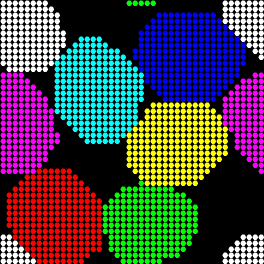(i) Select an integer q in the range 1 through 7. Cells may be in any of the states 1 through q (represented by q distinct colors) and do not change their state as the system evolves. (ii) Select a grid size s and an integer n such that 1 <= n <= s (this is the "selection range"). (iii) Select a real-valued "concentration" c (range 0 through 1), which is the probability that a location on the grid is occupied by a cell, then set up the initial state of the system by placing c.s2 cells at random locations on the grid and in random states subject to the condition that there are approximately equal numbers of cells in each of the q states. (iv) Select an integer m in the range 1 through 9999 (the "lossy move chance"). (v) The system evolves as a succession of "steps", each of which consists of a succession of s2 "substeps". (vi) A "substep" consists of the following process: (a) Select at random a cell which is not surrounded by 8 neighbors all in the same state. (b) Among those locations on the grid that are within n units of distance from the cell select one at random (which is not the location of the cell itself). (Distance is measured as the length of a minimal path, in horizontal and vertical steps, along the grid.) (c) If there is a cell at that location and either it has the same state as the first or it is surrounded by 8 neighbors all in the same state as that (second) cell then continue with the next substep. (d) If there is no cell at that location then calculate the "gain" g, that is, the increase or decrease in the number of neighbors of the cell in the same state as that cell, which would result from moving the cell to that location. If g >= 0 then move the cell to the new location. If g = -1 then make the move with a probability of 1 in m. (e) If there is a cell at that location then calculate the combined "gain" g, that is, the net increase or decrease in the number of neighbors of the two cells in the same state as those cells which would result from swapping them. If g >= 0 then swap the cells. If g = -1 or g = -2 then perform the swap with a probability of 1 in m. (f) This completes a substep.

The rule allowing a move or a swap which results in a loss, although seldom occurring, makes this algorithm a simple relative of the Metropolis and Glauber dynamics algorithms used in computational physics.

### Viral Replication

The rules for this automaton are as follows: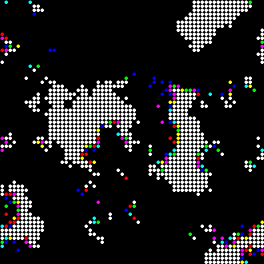(i) Select an integer q in the range 2 through 254. Cells may be in any of the states 1 through q. A cell in state q is said to be "healthy", a cell in state 1 is "fully infected" and a cell in any other state is "infected". (ii) Select a grid size s. (iii) Select integers k1 (range 0 through 100), k2 (range 0 through 9999) and k3 (range 0 through 100), interpreted respectively as specifying the active infection rate, the base rate and the chance of cell division as described above. (iv) Set up the initial state of the system by placing healthy cells at random locations on the grid so that approximately half of the locations are occupied by cells. (v) The system evolves as a succession of "steps", each of which consists of a succession of s2 "substeps". (vi) A "substep" consists of the following process: (a) Select a random location. If it is not occupied by a cell then this substep is completed. (b) Otherwise if the cell at this location is healthy then its state changes to q-1 (so it becomes infected) with probability k2/100,000. (c) If the cell does not become infected, and at least one of the eight neighboring locations is empty, the cell divides with probability k3/100, with one daughter cell remaining at this location and the other occupying one of the empty neighboring locations (chosen at random). (d) If the cell at this location is infected but not fully infected then its state decreases by 1. (e) If the cell at this location is fully infected then it disappears, and the state of each neighboring cell which is not fully infected decreases by 1 with probability k1/100. (f) This completes a substep.

### Diffusion-Limited Aggregation

The rules for this automaton are as follows: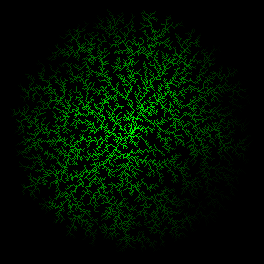(i) Select an integer q which is one of 2, 4, 8 or 64. Cells may be in any of the states 1 through q. Cells are either "fixed" or "mobile". (ii) Select a grid size s. (iii) Select integers k1 (range 5 through 100) and k2 (range 1 through minimum of s and 250), interpreted respectively as specifying the initial percentage concentration of cells on the grid and the number of seed cells. (iv) Set up the initial state of the system by placing (a) k2 "seed" cells either at random locations on the grid or at certain predetermined positions and (b) the remaining cells (whose number is determined by k1 and the grid size) at random empty locations. The seed cells are all fixed and the other cells are all mobile. The seed cells are all in state q (and are usually colored white) and the other cells are all in state 1 (and are invisible). (v) The system evolves as a succession of "steps", each of which consists of a succession of s2 "substeps". (vi) A "substep" consists of the following process: (a) Select a random location. If there is no mobile cell at this location then this substep is completed. (b) If one of the neighboring locations has a fixed cell then this mobile cell changes to a fixed cell, and is assigned a state in the range 2 through q and an appropriate color (this completes this substep). (c) If no neighboring location has a fixed cell then this mobile cell moves at random to one of the empty neighboring locations (if any). (d) If the new location of the cell is beyond the circular region centered on the central location with radius s/2 then the cell ceases to exist. (f) This completes a substep.